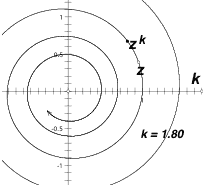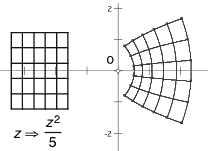# Graphing Complex Curves as Loci

Once we have access to a toolbox, we can begin modeling in earnest. Though the toolbox only gives us single operations that are defined on points (for example: addition, defined to map two addend points to a third sum point), two general operations allow us to extend these productively:

Concatenation of Operations. By using different tools sequentially, we can build more detailed expressions out of primitive operations; or (the reverse) we can decompose tangled expressions into simple sequences of primitives. This is straightforward "order-of-operations"-type thinking. To construct (2zA)2/B from points z, A, and B, we might first use the addition tool to double z, then the subtraction tool (for 2z A), then a multiplication—(2zA)2—and finally a division (by B). The tools of the Complex Arithmetic toolbox label their produced points with an appropriate symbolic expression stated in terms of the labels of the input points (as in Figure 1). These labels—combined with judicious hiding of intermediate results when they are no longer necessary—help track the arithmetic interpretation of the growing total expression, as we develop its parts with individual tools.

Graphing by Locus Construction. While so far we have worked only with points, the Locus command allows us to visualize—or "image"—any planar curve or curves under a complex transformation, by constructing the locus of a transformed point as a function of the motion of its pre-image point traveling along the pre-image curve. This is a straightforward application of the locus interpretation of graphing used on the Cartesian plane to first conceptualize graphs of real functions: if a given pre-image point x defines, through its position on the horizontal axis, some image point (x, f(x)), then the locus of the image point as the pre-image travels the horizontal axis is the total graph y = f(x). Here, if a given pre-image point z defines an image z' under some transformation, then the locus of z' as z travels some curve s is the image s' of that curve. With this technique, Sketchpad's Locus command can construct the image of segments, rays, lines, circles, polygon perimeters, arcs and their segments and sectors, and finally, function plots and other loci.

These two operations combine to provide a general-purpose complex graphing facility, as demonstrated in Figure 5. In the left illustration, two draggable points—z and k—define a third point zk. The spiral graph—of z raised to all real powers—is the locus of zk as k travels the real axis. Dragging point k in the completed diagram simply slides zk along the spiral; dragging point z (which is free in the plane) expands and contracts the spiral's curvature. This example, as well as that of Figure 5b, also demonstrates the conventional solution to what might be called "the problem of complex graphing." When graphing R-to-R functions on a Cartesian coordinate system, the one-dimensional output range—f(x)—is projected perpendicular to the one-dimensional input domain x, creating a two-dimensional graph. But with complex functions, both input and output values are already two-dimensional (real and imaginary), and thus four dimensions are required to visualize the result. The approach commonly taken for textbook illustrations is to graph only some subset of the input plane, and to superimpose its output on the same coordinate system. Usually these examples adopt the language of transformations (a transformed image is superimposed on its own pre-image), and a frequent choice for pre-image is some sort of checkerboard or lattice, since its abundant interior right angles allow one to quickly inspect conformality. In Sketchpad, the pre-image lattice in Figure 5b is constructed with Euclidean segments. Complex arithmetic tools produce a point z'  = z2/5 given some independent point z. (These points are no longer visible in the illustration.) The image lattice is then constructed curved segment by curved segment, where each curved segment is the locus of point z' as point z travels the corresponding straight segment in the pre-image lattice.Figure 5a. Point zk is z raised to the present value (1.8) of k; the spiral depicts z raised to all real powers.Figure 5b. A lattice and its image under the given transformation.

It is only at this point—that is, only once these conceptual and technological tools are established—that applying a dynamic geometry environment to complex functions becomes uniquely interesting. Other mathematics packages can compute complex values and graph complex functions with less conceptual overhead than Sketchpad, but they lack dynamic geometry's signature ability to experiment with a configuration interactively by dragging its components. Dragging and—to a lesser degree—animation allow one not only to visualize these systems across change or parametric evolution, but permit one to following Needham's (1997) seminal work in bringing a kinematic, Newton-like approach of "geometric calculus" to complex analysis and visualization.Figure 6. Winding about roots.

Figure 6 suggests some of the visual dynamics that become accessible in the dragging environment. Each picture shows a circle being dragged across the plane, and the image of that circle under the function

f(z) = (z r1)(zr2)(zr3)(z r4)

As usual, the image was constructed by first calculating—using the complex arithmetic tools—the function's value f(z) for one independent point z, and then constructing the locus of f(z) as z defined (or traveled around) the pre-image circle.

Over the four frames (read from top to bottom), the user drags and expands the circle to encircle roots of the function. In the first frame, only r1 is "captured." Correspondingly, the circle's image winds around the origin, since the origin (zero) is the image of the root. But as the circle grows (frame 2) to capture a second root (r2 in frame 3), an inner loop of the image snakes out to encircle the origin a second time. In the final frame, the circle expands toward r3, and we see a new loop forming to wind a third time around the origin. The winding number of the image determines the number of roots (counted in their algebraic multiplicity) encircled by the pre-image.

As is usually the case with descriptions of dynamic geometry, actually dragging these configurations is much more compelling than observing static pictures!